# High School Math : Right Triangles

## Example Questions

### Example Question #1 : Triangles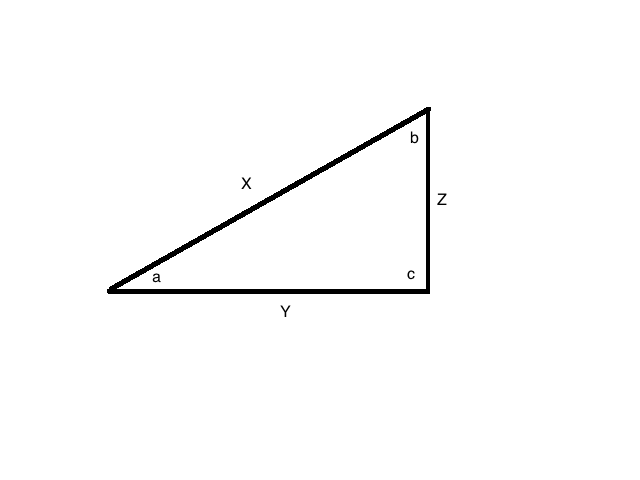In this figure, side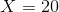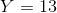, and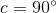. What is the value of angle?

Undefined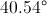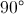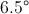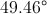Explanation:

Since, we know we are working with a right triangle.

That means that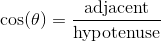.

In this problem, that would be: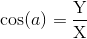Plug in our given values: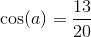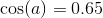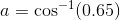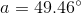### Example Question #2 : Triangles

Let ABC be a right triangle with sides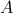= 3 inches,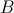= 4 inches, and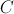= 5 inches. In degrees, what is the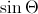where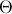is the angle opposite of side?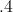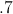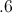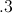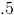Explanation: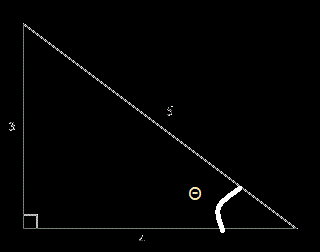We are looking for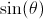. Remember the definition of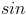in a right triangle is the length of the opposite side divided by the length of the hypotenuse.

So therefore, without figuring outwe can find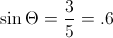### Example Question #3 : TrianglesIn this figure, if angle, side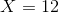, and side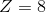, what is the measure of angle?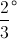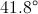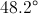UndefinedExplanation:

Since, we know we are working with a right triangle.

That means that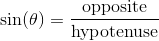.

In this problem, that would be: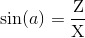Plug in our given values: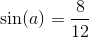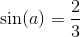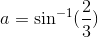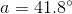### Example Question #1 : Understanding 30 60 90 TrianglesIn this figure,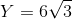, and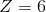. What is the value of angle?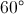Undefined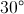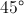Explanation:

Notice that these sides fit the pattern of a 30:60:90 right triangle: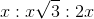.

In this case,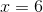.

Since angleis opposite, it must be.

### Example Question #2 : Understanding 30 60 90 Triangles

A triangle has angles of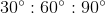. If the side opposite theangle is, what is the length of the side opposite?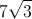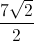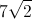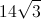The pattern foris that the sides will be.
If the side oppositeis, then the side oppositewill be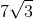.